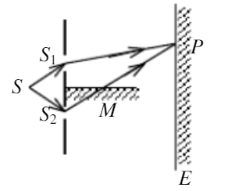$\text{A.}$ $P$ 点处仍为明条纹. $\text{B.}$ $P$ 点处为暗条纹. $\text{C.}$ 不能确定 $P$ 点处是明条纹还是暗条纹. $\text{D.}$ 无干涉条纹.